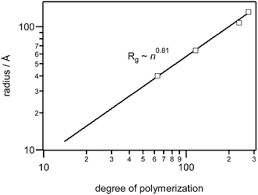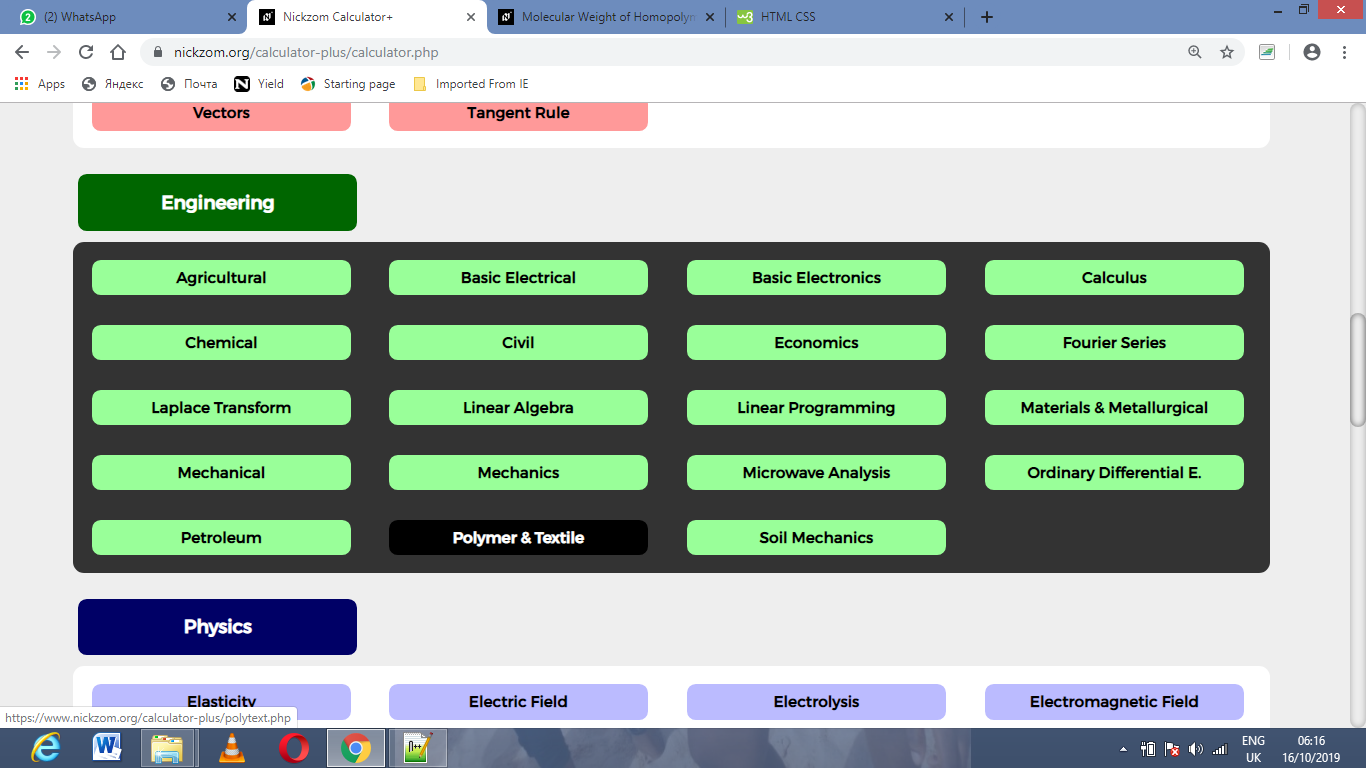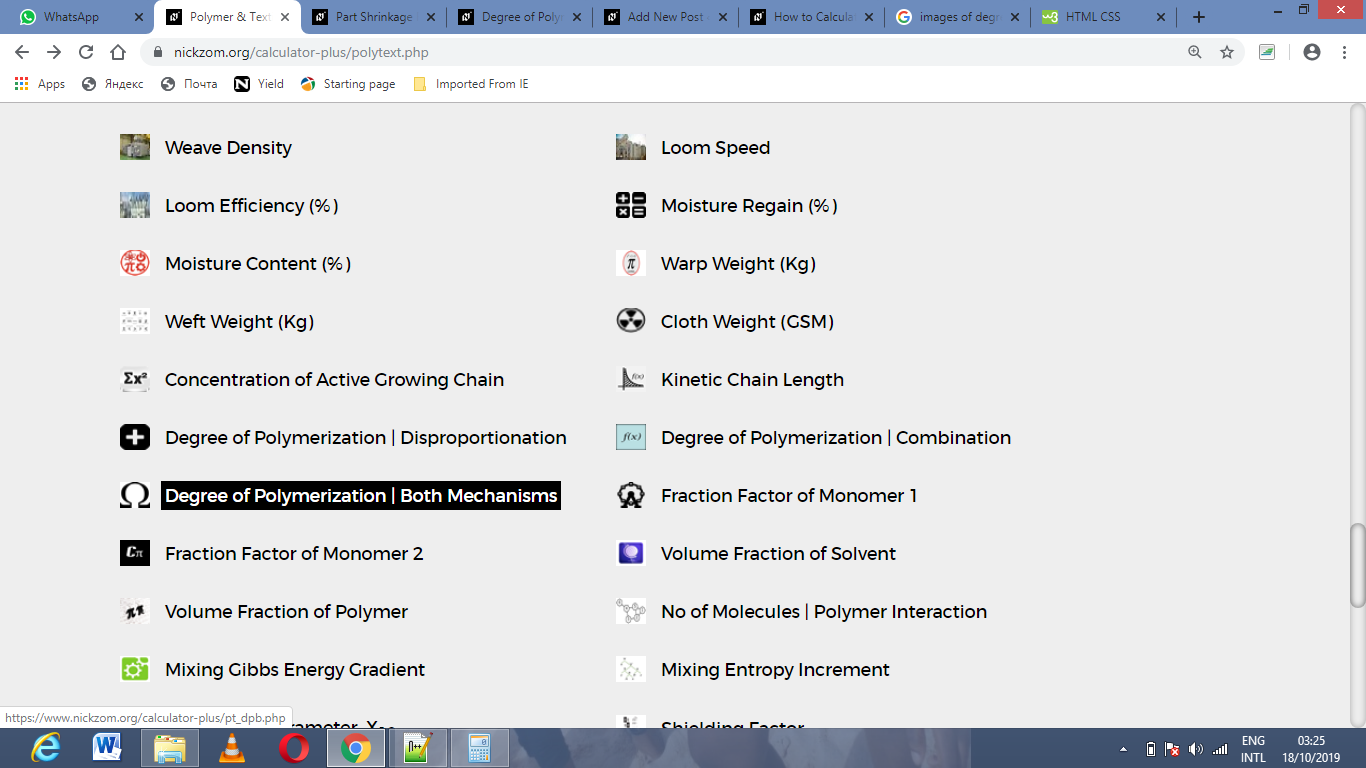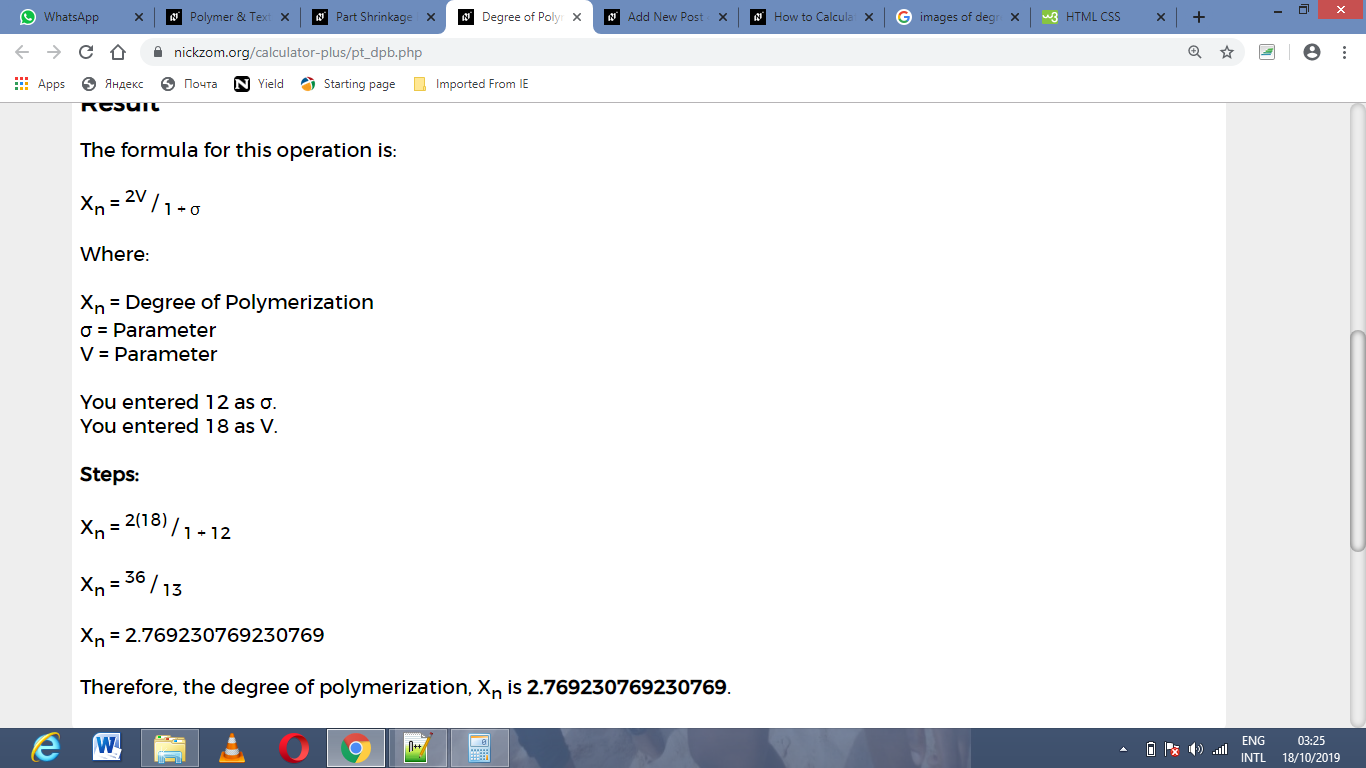# How to Calculate and Solve for Degree of Polymerization | Both Mechanisms | Polymer & TextileThe image above represents degree of polymerization | both mechanisms.

To compute for degree of polymerization, two essential parameters are needed and these parameters are parameter (σ) and parameter (V).

The formula for calculating the degree of polymerization:

Xn = 2V / 1 + σ

Where:

Xn = Degree of Polymerization
σ = Parameter
V = Parameter

Let’s solve an example;
Find the degree of polymerization when the parameter is 12 and the parameter is 18.

This implies that;

σ = Parameter = 12
V = Parameter = 18

Xn = 2V / 1 + σ
Xn = 2(18) / 1 + 12
Xn = 36 / 13
Xn = 2.769

Therefore, the degree of polymerization is 2.769.

Calculating the Parameter when the Degree of Polymerization and Parameter is Given.

V = Xn (1 + σ) / 2

Where:

V = Parameter
σ = Parameter
Xn = Degree of Polymerization

Let’s solve an example;
Find the parameter when the degree of polymerization is 22 and the parameter is 4.

This implies that;

Xn = Degree of Polymerization = 22
σ = Parameter = 4

V = Xn (1 + σ) / 2
V = 22 (1 + 4) / 2
V = 22 (5) / 2
V = 110 / 2
V = 55

Therefore, the parameter is 55.

Calculating the Parameter when the Degree of Polymerization and Parameter is Given.

σ = 2V / Xn – 1

Where:

σ = Parameter
V = Parameter
Xn = Degree of Polymerization

Let’s solve an example;
Find the parameter when the degree of polymerization is 32 and the parameter is 14.

This implies that;

Xn = Degree of Polymerization = 32
V = Parameter = 14

σ = 2V / Xn – 1
σ = 2(14) / 32 – 1
σ = 28 / 32 – 1
σ = 0.875 – 1
σ = -0.125

Therefore, the parameter is -0.125.

Nickzom Calculator – The Calculator Encyclopedia is capable of calculating the degree of polymerization | both mechanisms.

To get the answer and workings of the degree of polymerization | both mechanisms using the Nickzom Calculator – The Calculator Encyclopedia. First, you need to obtain the app.

You can get this app via any of these means:

To get access to the professional version via web, you need to register and subscribe for NGN 1,500 per annum to have utter access to all functionalities.
You can also try the demo version via https://www.nickzom.org/calculator

Apple (Paid) – https://itunes.apple.com/us/app/nickzom-calculator/id1331162702?mt=8
Once, you have obtained the calculator encyclopedia app, proceed to the Calculator Map, then click on Polymer & Textile under Engineering.Now, Click on Degree of Polymerization | Both Mechanisms under Polymer & TextileThe screenshot below displays the page or activity to enter your values, to get the answer for the degree of polymerization according to the respective parameters which is the parameter (σ) and parameter (V).Now, enter the values appropriately and accordingly for the parameters as required by the parameter (σ) is 12 and parameter (V) is 18.Finally, Click on CalculateAs you can see from the screenshot above, Nickzom Calculator– The Calculator Encyclopedia solves for the degree of polymerization | both mechanisms and presents the formula, workings and steps too.